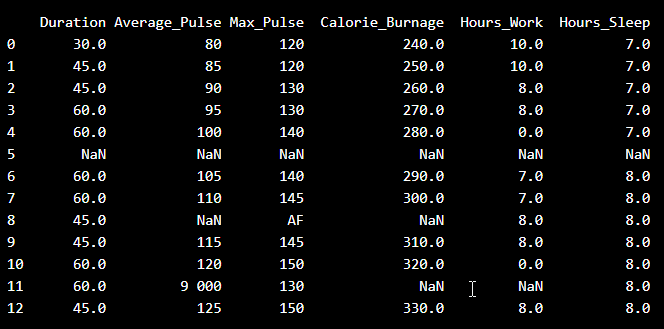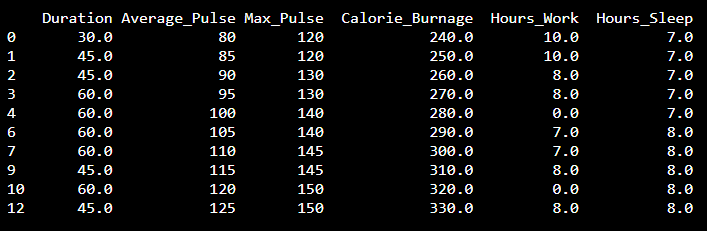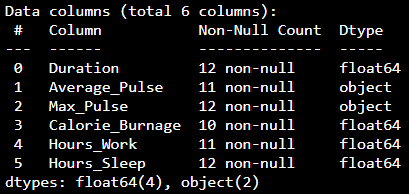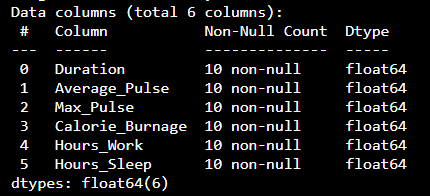# Data Science - Data Preparation

Before analyzing data, a Data Scientist must extract the data, and make it clean and valuable.

## Extract and Read Data With Pandas

Before data can be analyzed, it must be imported/extracted.

In the example below, we show you how to import data using Pandas in Python.

We use the `read_csv()` function to import a CSV file with the health data:

### Example

import pandas as pd

print(health_data)
Try it Yourself »

### Example Explained

• Import the Pandas library
• Name the data frame as `health_data`.
• `header=0` means that the headers for the variable names are to be found in the first row (note that 0 means the first row in Python)
• `sep=","` means that "," is used as the separator between the values. This is because we are using the file type .csv (comma separated values)

Tip: If you have a large CSV file, you can use the ``` head()``` function to only show the top 5rows:

### Example

import pandas as pd

Try it Yourself »

## Data Cleaning

Look at the imported data. As you can see, the data are "dirty" with wrongly or unregistered values:• There are some blank fields
• Average pulse of 9 000 is not possible
• 9 000 will be treated as non-numeric, because of the space separator
• One observation of max pulse is denoted as "AF", which does not make sense

So, we must clean the data in order to perform the analysis.

## Remove Blank Rows

We see that the non-numeric values (9 000 and AF) are in the same rows with missing values.

Solution: We can remove the rows with missing observations to fix this problem.

When we load a data set using Pandas, all blank cells are automatically converted into "NaN" values.

So, removing the NaN cells gives us a clean data set that can be analyzed.

We can use the `dropna()` function to remove the NaNs. axis=0 means that we want to remove all rows that have a NaN value:

### Example

health_data.dropna(axis=0,inplace=True)

print(health_data)
Try it Yourself »

The result is a data set without NaN rows:## Data Categories

To analyze data, we also need to know the types of data we are dealing with.

Data can be split into three main categories:

1. Numerical - Contains numerical values. Can be divided into two categories:
• Discrete: Numbers are counted as "whole". Example: You cannot have trained 2.5 sessions, it is either 2 or 3
• Continuous: Numbers can be of infinite precision. For example, you can sleep for 7 hours, 30 minutes and 20 seconds, or 7.533 hours
2. Categorical - Contains values that cannot be measured up against each other. Example: A color or a type of training
3. Ordinal - Contains categorical data that can be measured up against each other. Example: School grades where A is better than B and so on

By knowing the type of your data, you will be able to know what technique to use when analyzing them.

## Data Types

We can use the `info()` function to list the data types within our data set:

### Example

print(health_data.info())
Try it Yourself »

Result:We see that this data set has two different types of data:

• Float64
• Object

We cannot use objects to calculate and perform analysis here. We must convert the type object to float64 (float64 is a number with a decimal in Python).

We can use the `astype()` function to convert the data into float64.

The following example converts "Average_Pulse" and "Max_Pulse" into data type float64 (the other variables are already of data type float64):

### Example

health_data["Average_Pulse"] = health_data['Average_Pulse'].astype(float)
health_data["Max_Pulse"] = health_data["Max_Pulse"].astype(float)

print (health_data.info())
Try it Yourself »

Result:Now, the data set has only float64 data types.

## Analyze the Data

When we have cleaned the data set, we can start analyzing the data.

We can use the `describe()` function in Python to summarize data:

### Example

print(health_data.describe())
Try it Yourself »

Result:

Duration Average_Pulse Max_Pulse Calorie_Burnage Hours_Work Hours_Sleep
Count 10.0 10.0 10.0 10.0 10.0 10.0
Mean 51.0 102.5 137.0 285.0 6.6 7.5
Std 10.49 15.4 11.35 30.28 3.63 0.53
Min 30.0 80.0 120.0 240.0 0.0 7.0
25% 45.0 91.25 130.0 262.5 7.0 7.0
50% 52.5 102.5 140.0 285.0 8.0 7.5
75% 60.0 113.75 145.0 307.5 8.0 8.0
Max 60.0 125.0 150.0 330.0 10.0 8.0
• Count - Counts the number of observations
• Mean - The average value
• Std - Standard deviation (explained in the statistics chapter)
• Min - The lowest value
• 25%, 50% and 75% are percentiles (explained in the statistics chapter)
• Max - The highest value

W3Schools is optimized for learning and training. Examples might be simplified to improve reading and learning. Tutorials, references, and examples are constantly reviewed to avoid errors, but we cannot warrant full correctness of all content. While using W3Schools, you agree to have read and accepted our terms of use, cookie and privacy policy.